## 4.4 The Second Derivative Test and Curve Sketching

### Learning Objectives

• Use concavity and inflection points to explain how the sign of the second derivative affects the shape of a function’s graph.
• Explain the concavity test for a function over an open interval.
• Explain the relationship between a function and its first and second derivatives.
• State the second derivative test for local extrema.
• Analyze a function and its derivatives to draw its graph.

# Concavity and Points of Inflection

We now know how to determine where a function is increasing or decreasing. However, there is another issue to consider regarding the shape of the graph of a function. If the graph curves, does it curve upward or curve downward? This notion is called the concavity of the function.

(Figure)(a) shows a function $f$ with a graph that curves upward. As $x$ increases, the slope of the tangent line increases. Thus, since the derivative increases as $x$ increases, $f^{\prime}$ is an increasing function. We say this function $f$ is concave up. (Figure)(b) shows a function $f$ that curves downward. As $x$ increases, the slope of the tangent line decreases. Since the derivative decreases as $x$ increases, $f^{\prime}$ is a decreasing function. We say this function $f$ is concave down.

### Definition

Let $f$ be a function that is differentiable over an open interval $I$. If $f^{\prime}$ is increasing over $I$, we say $f$ is concave up over $I$. If $f^{\prime}$ is decreasing over $I$, we say $f$ is concave down over $I$.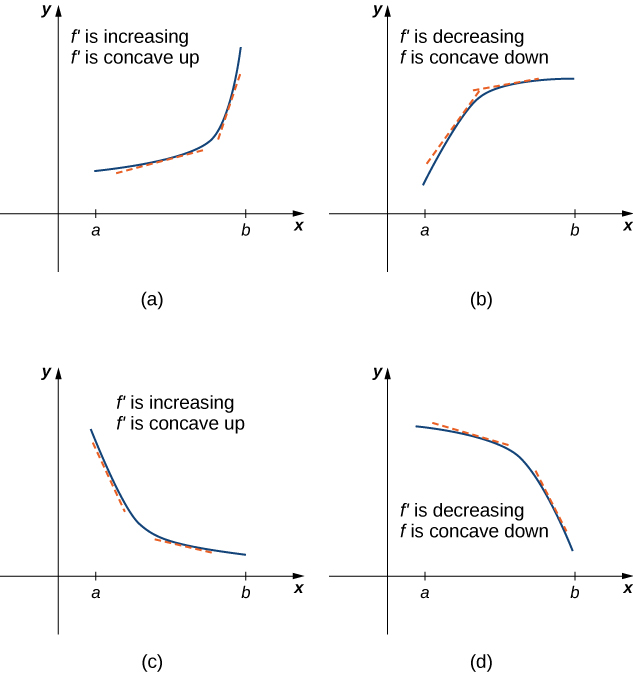Figure 5. (a), (c) Since $f^{\prime}$ is increasing over the interval $(a,b)$, we say $f$ is concave up over $(a,b)$. (b), (d) Since $f^{\prime}$ is decreasing over the interval $(a,b)$, we say $f$ is concave down over $(a,b)$.

In general, without having the graph of a function $f$, how can we determine its concavity? By definition, a function $f$ is concave up if $f^{\prime}$ is increasing. From Corollary 3, we know that if $f^{\prime}$ is a differentiable function, then $f^{\prime}$ is increasing if its derivative $f^{\prime \prime}(x)>0$. Therefore, a function $f$ that is twice differentiable is concave up when $f^{\prime \prime}(x)>0$. Similarly, a function $f$ is concave down if $f^{\prime}$ is decreasing. We know that a differentiable function $f^{\prime}$ is decreasing if its derivative $f^{\prime \prime}(x)<0$. Therefore, a twice-differentiable function $f$ is concave down when $f^{\prime \prime}(x)<0$. Applying this logic is known as the concavity test.

### Test for Concavity

Let $f$ be a function that is twice differentiable over an interval $I$.

1. If $f^{\prime \prime}(x)>0$ for all $x \in I$, then $f$ is concave up over $I$.
2. If $f^{\prime \prime}(x)<0$ for all $x \in I$, then $f$ is concave down over $I$.

We conclude that we can determine the concavity of a function $f$ by looking at the second derivative of $f$. In addition, we observe that a function $f$ can switch concavity ((Figure)). However, a continuous function can switch concavity only at a point $x$ if $f^{\prime \prime}(x)=0$ or $f^{\prime \prime}(x)$ is undefined. Consequently, to determine the intervals where a function $f$ is concave up and concave down, we look for those values of $x$ where $f^{\prime \prime}(x)=0$ or $f^{\prime \prime}(x)$ is undefined. When we have determined these points, we divide the domain of $f$ into smaller intervals and determine the sign of $f^{\prime \prime}$ over each of these smaller intervals. If $f^{\prime \prime}$ changes sign as we pass through a point $x$, then $f$ changes concavity. It is important to remember that a function $f$ may not change concavity at a point $x$ even if $f^{\prime \prime}(x)=0$ or $f^{\prime \prime}(x)$ is undefined. If, however, $f$ does change concavity at a point $a$ and $f$ is continuous at $a$, we say the point $(a,f(a))$ is an inflection point of $f$.

### Definition

If $f$ is continuous at $a$ and $f$ changes concavity at $a$, the point $(a,f(a))$ is an inflection point of $f$.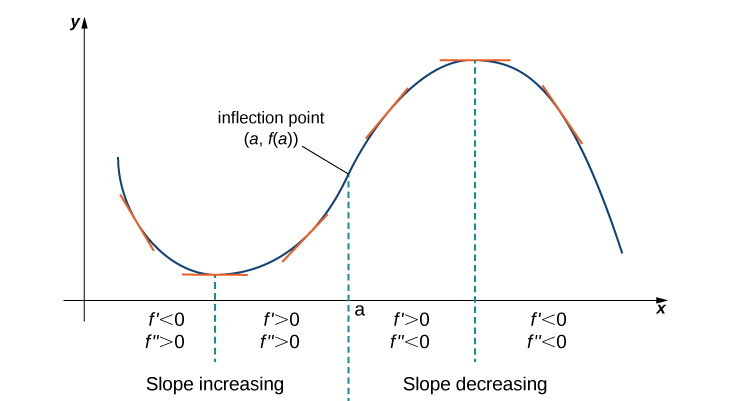Figure 6. Since $f^{\prime \prime}(x)>0$ for $x<a$, the function $f$ is concave up over the interval $(−\infty,a)$. Since $f^{\prime \prime}(x)<0$ for $x>a$, the function $f$ is concave down over the interval $(a,\infty)$. The point $(a,f(a))$ is an inflection point of $f$.

### Testing for Concavity

For the function $f(x)=x^3-6x^2+9x+30$, determine all intervals where $f$ is concave up and all intervals where $f$ is concave down. List all inflection points for $f$. Use a graphing utility to confirm your results.

For $f(x)=−x^3+\frac{3}{2}x^2+18x$, find all intervals where $f$ is concave up and all intervals where $f$ is concave down.

#### Hint

Find where $f^{\prime \prime}(x)=0$.

We now summarize, in (Figure), the information that the first and second derivatives of a function $f$ provide about the graph of $f$, and illustrate this information in (Figure).

What Derivatives Tell Us about Graphs
Sign of $f^{\prime}$ Sign of $f^{\prime \prime}$ Is $f$ increasing or decreasing? Concavity
Positive Positive Increasing Concave up
Positive Negative Increasing Concave down
Negative Positive Decreasing Concave up
Negative Negative Decreasing Concave down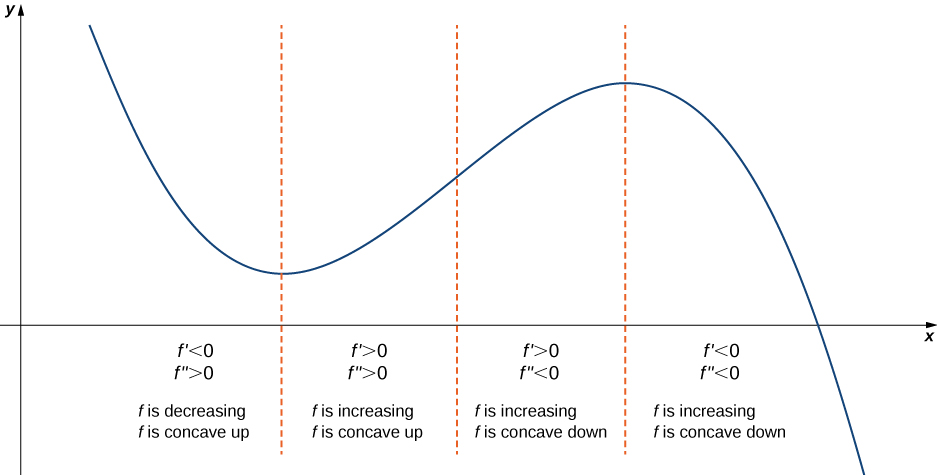Figure 8. Consider a twice-differentiable function $f$ over an open interval $I$. If $f^{\prime}(x)>0$ for all $x \in I$, the function is increasing over $I$. If $f^{\prime}(x)<0$ for all $x \in I$, the function is decreasing over $I$. If $f^{\prime \prime}(x)>0$ for all $x \in I$, the function is concave up. If $f^{\prime \prime}(x)<0$ for all $x \in I$, the function is concave down on $I$.

# The Second Derivative Test

The first derivative test provides an analytical tool for finding local extrema, but the second derivative can also be used to locate extreme values. Using the second derivative can sometimes be a simpler method than using the first derivative.

We know that if a continuous function has a local extrema, it must occur at a critical point. However, a function need not have a local extrema at a critical point. Here we examine how the second derivative test can be used to determine whether a function has a local extremum at a critical point. Let $f$ be a twice-differentiable function such that $f^{\prime}(a)=0$ and $f^{\prime \prime}$ is continuous over an open interval $I$ containing $a$. Suppose $f^{\prime \prime}(a)<0$. Since $f^{\prime \prime}$ is continuous over $I$, $f^{\prime \prime}(x)<0$ for all $x \in I$ ((Figure)). Then, by Corollary 3, $f^{\prime}$ is a decreasing function over $I$. Since $f^{\prime}(a)=0$, we conclude that for all $x \in I, \, f^{\prime}(x)>0$ if $x<a$ and $f^{\prime}(x)<0$ if $x>a$. Therefore, by the first derivative test, $f$ has a local maximum at $x=a$. On the other hand, suppose there exists a point $b$ such that $f^{\prime}(b)=0$ but $f^{\prime \prime}(b)>0$. Since $f^{\prime \prime}$ is continuous over an open interval $I$ containing $b$, then $f^{\prime \prime}(x)>0$ for all $x \in I$ ((Figure)). Then, by Corollary $3, \, f^{\prime}$ is an increasing function over $I$. Since $f^{\prime}(b)=0$, we conclude that for all $x \in I$, $f^{\prime}(x)<0$ if $x<b$ and $f^{\prime}(x)>0$ if $x>b$. Therefore, by the first derivative test, $f$ has a local minimum at $x=b$.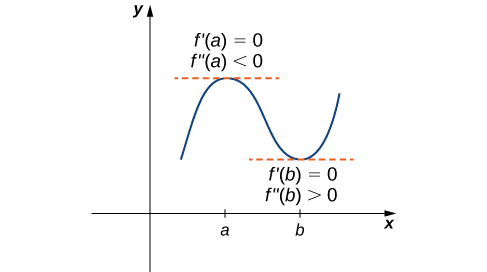Figure 9. Consider a twice-differentiable function $f$ such that $f^{\prime \prime}$ is continuous. Since $f^{\prime}(a)=0$ and $f^{\prime \prime}(a)<0$, there is an interval $I$ containing $a$ such that for all $x$ in $I$, $f$ is increasing if $x<a$ and $f$ is decreasing if $x>a$. As a result, $f$ has a local maximum at $x=a$. Since $f^{\prime}(b)=0$ and $f^{\prime \prime}(b)>0$, there is an interval $I$ containing $b$ such that for all $x$ in $I$, $f$ is decreasing if $x<b$ and $f$ is increasing if $x>b$. As a result, $f$ has a local minimum at $x=b$.

### Second Derivative Test

Suppose $f^{\prime}(c)=0, \, f^{\prime \prime}$ is continuous over an interval containing $c$.

1. If $f^{\prime \prime}(c)>0$, then $f$ has a local minimum at $c$.
2. If $f^{\prime \prime}(c)<0$, then $f$ has a local maximum at $c$.
3. If $f^{\prime \prime}(c)=0$, then the test is inconclusive.

Note that for case iii. when $f^{\prime \prime}(c)=0$, then $f$ may have a local maximum, local minimum, or neither at $c$. For example, the functions $f(x)=x^3$, $f(x)=x^4$, and $f(x)=−x^4$ all have critical points at $x=0$. In each case, the second derivative is zero at $x=0$. However, the function $f(x)=x^4$ has a local minimum at $x=0$ whereas the function $f(x)=−x^4$ has a local maximum at $x=0$ and the function $f(x)=x^3$ does not have a local extremum at $x=0$.

Let’s now look at how to use the second derivative test to determine whether $f$ has a local maximum or local minimum at a critical point $c$ where $f^{\prime}(c)=0$.

### Using the Second Derivative Test

Use the second derivative to find the location of all local extrema for $f(x)=x^5-5x^3$.

Consider the function $f(x)=x^3-(\frac{3}{2})x^2-18x$. The points $c=3,-2$ satisfy $f^{\prime}(c)=0$. Use the second derivative test to determine whether $f$ has a local maximum or local minimum at those points.

#### Hint

$f^{\prime \prime}(x)=6x-3$

We have now developed the tools we need to determine where a function is increasing and decreasing, as well as acquired an understanding of the basic shape of the graph. Next we discuss what happens to a function as $x \to \pm \infty$. At that point, we have enough tools to provide accurate graphs of a large variety of functions.

# Guidelines for Drawing the Graph of a Function

We now have enough analytical tools to draw graphs of a wide variety of algebraic and transcendental functions. Before showing how to graph specific functions, let’s look at a general strategy to use when graphing any function.

### Problem-Solving Strategy: Drawing the Graph of a Function

Given a function $f$ use the following steps to sketch a graph of $f$:

1. Determine the domain of the function.
2. Locate the $x$– and $y$-intercepts.
3. Evaluate $\underset{x\to \infty }{\lim}f(x)$ and $\underset{x\to −\infty }{\lim}f(x)$ to determine the end behavior. If either of these limits is a finite number $L$, then $y=L$ is a horizontal asymptote. If either of these limits is $\infty$ or $−\infty$, determine whether $f$ has an oblique asymptote. If $f$ is a rational function such that $f(x)=\frac{p(x)}{q(x)}$, where the degree of the numerator is greater than the degree of the denominator, then $f$ can be written as
$f(x)=\frac{p(x)}{q(x)}=g(x)+\frac{r(x)}{q(x)}$,

where the degree of $r(x)$ is less than the degree of $q(x)$. The values of $f(x)$ approach the values of $g(x)$ as $x\to \pm \infty$. If $g(x)$ is a linear function, it is known as an oblique asymptote.

4. Determine whether $f$ has any vertical asymptotes.
5. Calculate $f^{\prime}$. Find all critical points and determine the intervals where $f$ is increasing and where $f$ is decreasing. Determine whether $f$ has any local extrema.
6. Calculate $f^{\prime \prime}$. Determine the intervals where $f$ is concave up and where $f$ is concave down. Use this information to determine whether $f$ has any inflection points. The second derivative can also be used as an alternate means to determine or verify that $f$ has a local extremum at a critical point.

Now let’s use this strategy to graph several different functions. We start by graphing a polynomial function.

### Sketching a Graph of a Polynomial

Sketch a graph of $f(x)=(x-1)^2 (x+2)$.

Sketch a graph of $f(x)=(x-1)^3 (x+2)$.

#### Hint

$f$ is a fourth-degree polynomial.

### Sketching a Rational Function

Sketch the graph of $f(x)=\frac{x^2}{1-x^2}$.

Sketch a graph of $f(x)=\frac{3x+5}{8+4x}$.

#### Hint

A line $y=L$ is a horizontal asymptote of $f$ if the limit as $x\to \infty$ or the limit as $x\to −\infty$ of $f(x)$ is $L$. A line $x=a$ is a vertical asymptote if at least one of the one-sided limits of $f$ as $x\to a$ is $\infty$ or $−\infty$.

### Sketching a Rational Function with an Oblique Asymptote

Sketch the graph of $f(x)=\frac{x^2}{x-1}$

Find the oblique asymptote for $f(x)=\frac{3x^3-2x+1}{2x^2-4}$.

#### Hint

Use long division of polynomials.

### Sketching the Graph of a Function with a Cusp

Sketch a graph of $f(x)=(x-1)^{2/3}$.

Consider the function $f(x)=5-x^{2/3}$. Determine the point on the graph where a cusp is located. Determine the end behavior of $f$.

#### Hint

A function $f$ has a cusp at a point $a$ if $f(a)$ exists, $f^{\prime}(a)$ is undefined, one of the one-sided limits as $x\to a$ of $f^{\prime}(x)$ is $+\infty$, and the other one-sided limit is $−\infty$.

### Key Concepts

• If $f^{\prime \prime}(x)>0$ over an interval $I$, then $f$ is concave up over $I$.
• If $f^{\prime \prime}(x)<0$ over an interval $I$, then $f$ is concave down over $I$.
• If $f^{\prime}(c)=0$ and $f^{\prime \prime}(c)>0$, then $f$ has a local minimum at $c$.
• If $f^{\prime}(c)=0$ and $f^{\prime \prime}(c)<0$, then $f$ has a local maximum at $c$.
• If $f^{\prime}(c)=0$ and $f^{\prime \prime}(c)=0$, then evaluate $f^{\prime}(x)$ at a test point $x$ to the left of $c$ and a test point $x$ to the right of $c$, to determine whether $f$ has a local extremum at $c$.
• For a polynomial function $p(x)=a_n x^n + a_{n-1} x^{n-1} + \cdots + a_1 x + a_0$, where $a_n \ne 0$, the end behavior is determined by the leading term $a_n x^n$. If $n\ne 0$, $p(x)$ approaches $\infty$ or $−\infty$ at each end.
• For a rational function $f(x)=\frac{p(x)}{q(x)}$, the end behavior is determined by the relationship between the degree of $p$ and the degree of $q$. If the degree of $p$ is less than the degree of $q$, the line $y=0$ is a horizontal asymptote for $f$. If the degree of $p$ is equal to the degree of $q$, then the line $y=\frac{a_n}{b_n}$ is a horizontal asymptote, where $a_n$ and $b_n$ are the leading coefficients of $p$ and $q$, respectively. If the degree of $p$ is greater than the degree of $q$, then $f$ approaches $\infty$ or $−\infty$ at each end.

2. For the function $y=x^3$, is $x=0$ both an inflection point and a local maximum/minimum?

3. For the function $y=x^3$, is $x=0$ an inflection point?

4. Is it possible for a point $c$ to be both an inflection point and a local extrema of a twice differentiable function?

5. Why do you need continuity for the first derivative test? Come up with an example.

6. Explain whether a concave-down function has to cross $y=0$ for some value of $x$.

7. Explain whether a polynomial of degree 2 can have an inflection point.

For the following exercises, analyze the graphs of $f^{\prime}$, then list all inflection points and intervals $f$ that are concave up and concave down.

18.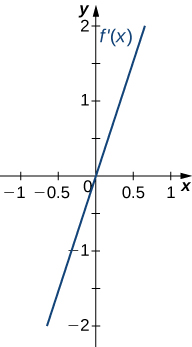19.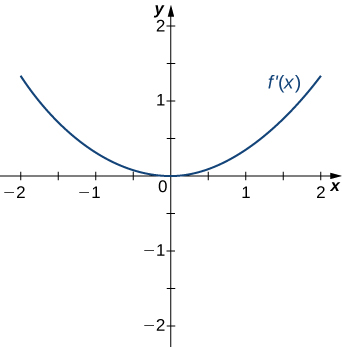20.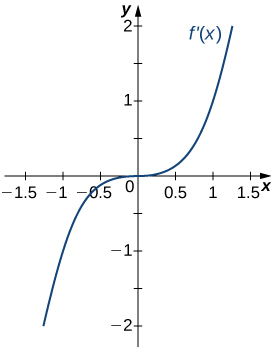21.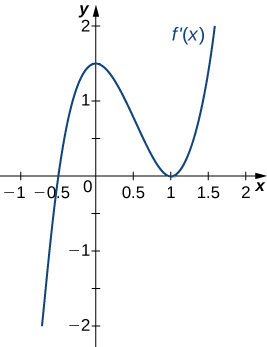22.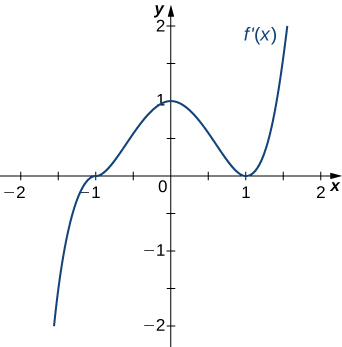For the following exercises, draw a graph that satisfies the given specifications for the domain $x=[-3,3]$. The function does not have to be continuous or differentiable.

23. $f(x)>0, \, f^{\prime}(x)>0$ over $x>1, \, -3<x<0, \, f^{\prime}(x)=0$ over $0<x<1$

24. $f^{\prime}(x)>0$ over $x>2, \, -3<x<-1, \, f^{\prime}(x)<0$ over $-1<x<2, \, f^{\prime \prime}(x)<0$ for all $x$

25. $f^{\prime \prime}(x)<0$ over $-1<x<1, \, f^{\prime \prime}(x)>0, \, -3<x<-1, \, 1<x<3$, local maximum at $x=0$, local minima at $x=\pm 2$

26. There is a local maximum at $x=2$, local minimum at $x=1$, and the graph is neither concave up nor concave down.

27. There are local maxima at $x=\pm 1$, the function is concave up for all $x$, and the function remains positive for all $x$.

For the following exercise, determine a. intervals where $f$ is concave up or concave down, and b. the inflection points of $f$.

30. $f(x)=x^3-4x^2+x+2$

For the following exercises, determine

1. intervals where $f$ is increasing or decreasing,
2. local minima and maxima of $f$,
3. intervals where $f$ is concave up and concave down, and
4. the inflection points of $f$.

31. $f(x)=x^2-6x$

32. $f(x)=x^3-6x^2$

33. $f(x)=x^4-6x^3$

34. $f(x)=x^{11}-6x^{10}$

35. $f(x)=x+x^2-x^3$

36. $f(x)=x^2+x+1$

37. $f(x)=x^3+x^4$

For the following exercises, determine

1. intervals where $f$ is increasing or decreasing,
2. local minima and maxima of $f$,
3. intervals where $f$ is concave up and concave down, and
4. the inflection points of $f$. Sketch the curve, then use a calculator to compare your answer. If you cannot determine the exact answer analytically, use a calculator.

38. [T] $f(x)= \sin (\pi x)- \cos (\pi x)$ over $x=[-1,1]$

39. [T] $f(x)=x + \sin (2x)$ over $x=[-\frac{\pi }{2},\frac{\pi }{2}]$

40. [T] $f(x)= \sin x+ \tan x$ over $(-\frac{\pi }{2},\frac{\pi }{2})$

41. [T] $f(x)=(x-2)^2 (x-4)^2$

42. [T] $f(x)=\frac{1}{1-x}, \, x \ne 1$

43. [T] $f(x)=\frac{\sin x}{x}$ over $x=[-2\pi ,0) \cup (0,2\pi]$

44. $f(x)= \sin x e^x$ over $x=[−\pi ,\pi]$

45. $f(x)=\ln x \sqrt{x}, \, x>0$

46. $f(x)=\frac{1}{4}\sqrt{x}+\frac{1}{x}, \, x>0$

47. $f(x)=\frac{e^x}{x}, \, x\ne 0$

For the following exercises, examine the graphs. Identify where the vertical asymptotes are located.

1.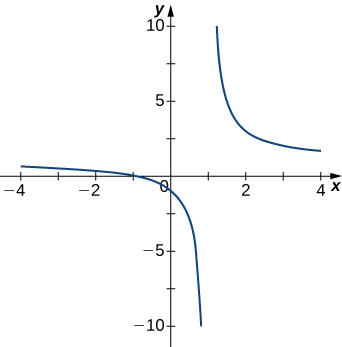2.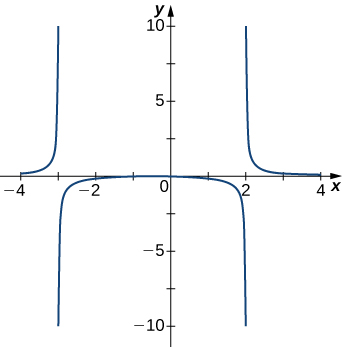3.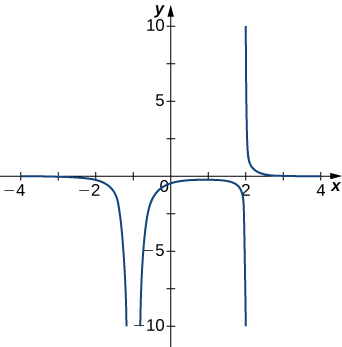4.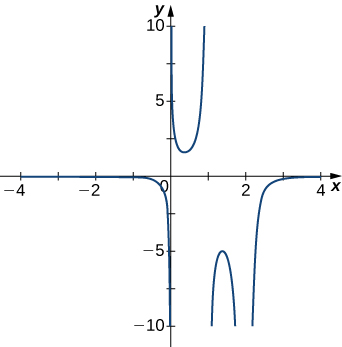5.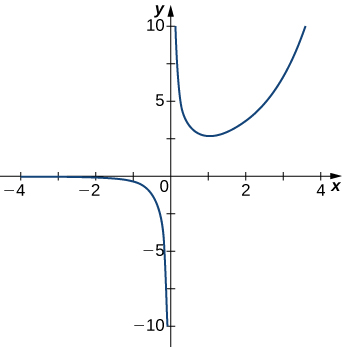For the following functions $f(x)$, determine whether there is an asymptote at $x=a$. Justify your answer without graphing on a calculator.

6. $f(x)=\frac{x+1}{x^2+5x+4}, \, a=-1$

7. $f(x)=\frac{x}{x-2}, \, a=2$

8. $f(x)=(x+2)^{3/2}, \, a=-2$

9. $f(x)=(x-1)^{-1/3}, \, a=1$

10. $f(x)=1+x^{-2/5}, \, a=1$

For the following exercises, construct a function $f(x)$ that has the given asymptotes.

11. $x=1$ and $y=2$

12. $x=1$ and $y=0$

13. $y=4$ and $x=-1$

14. $x=0$

For the following exercises, graph the function on a graphing calculator on the window $x=[-5,5]$ and estimate the horizontal asymptote or limit. Then, calculate the actual horizontal asymptote or limit.

15. [T] $f(x)=\frac{1}{x+10}$

16. [T] $f(x)=\frac{x+1}{x^2+7x+6}$

17. [T] $\underset{x\to −\infty }{\lim} x^2+10x+25$

18. [T] $\underset{x\to −\infty }{\lim}\frac{x+2}{x^2+7x+6}$

19. [T] $\underset{x\to \infty }{\lim}\frac{3x+2}{x+5}$

For the following exercises, draw a graph of the functions without using a calculator. Be sure to notice all important features of the graph: local maxima and minima, inflection points, and asymptotic behavior.

20. $y=3x^2+2x+4$

21. $y=x^3-3x^2+4$

22. $y=\frac{2x+1}{x^2+6x+5}$

23. $y=\frac{x^3+4x^2+3x}{3x+9}$

24. $y=\frac{x^2+x-2}{x^2-3x-4}$

25. $y=\sqrt{x^2-5x+4}$

26. $y=2x\sqrt{16-x^2}$

27. $y=\frac{ \cos x}{x}$, on $x=[-2\pi ,2\pi]$

28. $y=e^x-x^3$

29. $y=x \tan x, \, x=[−\pi ,\pi]$

30. $y=x \ln (x), \, x>0$

31. $y=x^2 \sin (x), \, x=[-2\pi ,2\pi]$

32. For $f(x)=\frac{P(x)}{Q(x)}$ to have an asymptote at $y=2$ then the polynomials $P(x)$ and $Q(x)$ must have what relation?

33. For $f(x)=\frac{P(x)}{Q(x)}$ to have an asymptote at $x=0$, then the polynomials $P(x)$ and $Q(x)$ must have what relation?

34. If $f^{\prime}(x)$ has asymptotes at $y=3$ and $x=1$, then $f(x)$ has what asymptotes?

35. Both $f(x)=\frac{1}{x-1}$ and $g(x)=\frac{1}{(x-1)^2}$ have asymptotes at $x=1$ and $y=0$. What is the most obvious difference between these two functions?

36. True or false: Every ratio of polynomials has vertical asymptotes.

## Glossary

end behavior
the behavior of a function as $x\to \infty$ and $x\to −\infty$
horizontal asymptote
if $\underset{x\to \infty }{\lim}f(x)=L$ or $\underset{x\to −\infty }{\lim}f(x)=L$, then $y=L$ is a horizontal asymptote of $f$
infinite limit at infinity
a function that becomes arbitrarily large as $x$ becomes large
limit at infinity
the limiting value, if it exists, of a function as $x\to \infty$ or $x\to −\infty$
oblique asymptote
the line $y=mx+b$ if $f(x)$ approaches it as $x\to \infty$ or $x\to −\infty$

### Key Concepts

• If $c$ is a critical point of $f$ and $f^{\prime}(x)>0$ for $x<c$ and $f^{\prime}(x)<0$ for $x>c$, then $f$ has a local maximum at $c$.
• If $c$ is a critical point of $f$ and $f^{\prime}(x)<0$ for $x<c$ and $f^{\prime}(x)>0$ for $x>c$, then $f$ has a local minimum at $c$.
• If $f^{\prime \prime}(x)>0$ over an interval $I$, then $f$ is concave up over $I$.
• If $f^{\prime \prime}(x)<0$ over an interval $I$, then $f$ is concave down over $I$.
• If $f^{\prime}(c)=0$ and $f^{\prime \prime}(c)>0$, then $f$ has a local minimum at $c$.
• If $f^{\prime}(c)=0$ and $f^{\prime \prime}(c)<0$, then $f$ has a local maximum at $c$.
• If $f^{\prime}(c)=0$ and $f^{\prime \prime}(c)=0$, then evaluate $f^{\prime}(x)$ at a test point $x$ to the left of $c$ and a test point $x$ to the right of $c$, to determine whether $f$ has a local extremum at $c$.